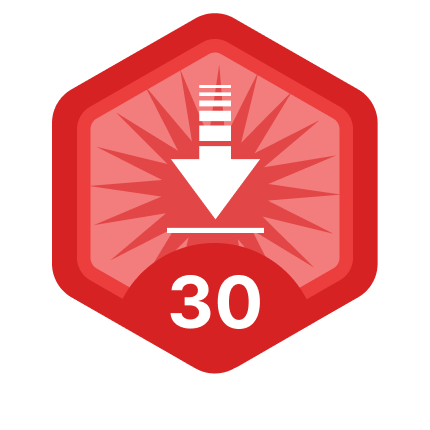Community Profile# Bill Whiten

### Retired

Last seen: 3 years ago Active since 2012

#### Statistics

All
••••••#### Content Feed

View by

Submitted

Probabilities for model comparison
Probability or probability distribution for comparing models or a model with repeat data.

Submitted

selectfields: Select specified field names from a struct
Selects fields listed in a cell array from a structSubmitted

Optional function arguments
Optional function arguments with default values: optndftsSubmitted

Functions istext iscelltext
Test for char or string variables; Test for cell array of char or string variables.

Submitted

nlsq & nnnlsq Least squares
Robust & non negative non linear least squares: nlsq & nnnlsqSubmitted

Equation selection using subset regression: regsubsets
Finds multiple linear regression equations relating measurement data to results. See regtest.htmlSubmitted

Weighted median (from data & sds) & its standard deviation
Interpolated weighted median with calculation of its standard deviation and distributionSubmitted

renamefields: Rename some field names in a struct
Changes struct field names from cell array list of old names to names in list of new namesSubmitted

Select points from graph: graphpoints
Get coordinates and indices of points from a graph [xx,yy,indx]=graphpoints(x,y)Submitted

Reconcile multiple examinations
Reconcile multiple examinations of multiple projectsSubmitted

EXPERT1
An easy to use deduction engine useful for small expert systems and embedding complex condition ...

Submitted

Boxplot: boxplotx without toolboxes
Boxplot: boxplotx Quartiles box, 1% whiskers, & outliersSubmitted

Expert2 Propositional Logic
A deduction engine useful for small expert systems and complex condition statements.Solved

Find the sum of all the numbers of the input vector
Find the sum of all the numbers of the input vector x. Examples: Input x = [1 2 3 5] Output y is 11 Input x ...

8 years ago

Submitted

KS statistic.zip
Test multiple samples for same distribution - probKSHow to change default path on startup for Mac?
You could try: http://www.mathworks.com/matlabcentral/fileexchange/39425-restore-project-status-for-selected-project Allows ...

9 years ago | 0

Submitted

nnls - Non negative least squares
nnls - Alternative to lsqnonneg: faster on large problems, improved convergence, optional restart

Submitted

Greyboxeval - Model quality evaluation
For models residues=model(data,parameters) with data sets at different experimental conditionsSubmitted

Greyboxbuild: complete a greybox model
Completes a model by relating model parameters to operating conditions using experimental dataSubmitted

linfitregsel - term selection in linear regression
Select terms in linear regression (equation fitting) using eigenvectors and term significanceLeast Squares Method- Νon linear to Linear
You can write the equation as f=(a/b)x-(1/b)fx and use linear regression for the coefficients (a/b) and (1/b). Convert these to ...

11 years ago | 0

Submitted

Restore project status for selected project
Restores search path and reopens editor files for selected folder/project during startup/restart